Introduction

Previously in this unit the graphs of power functions were presented. Radical equation graphs fall under the heading of power functions when the power is between the values of 0 and 1, in other words a fraction. Remember that rational exponents can be translated to roots. Therefore, the graph of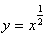is the same graph as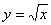. Similarly the graph of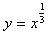is the same graph as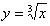.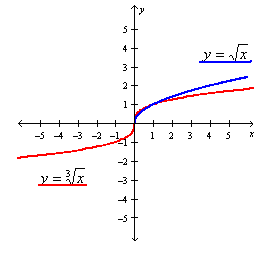Listen